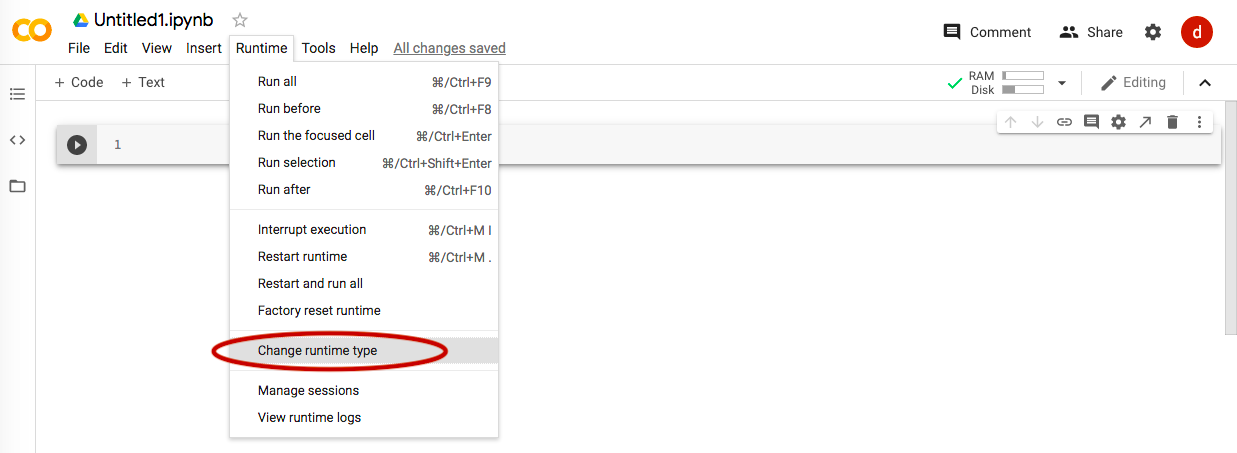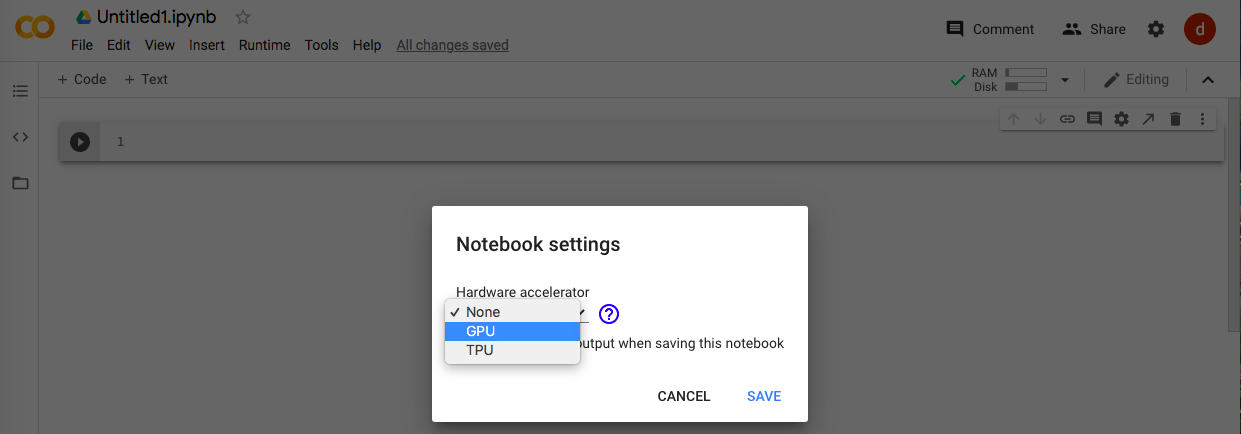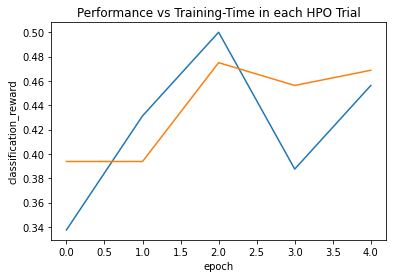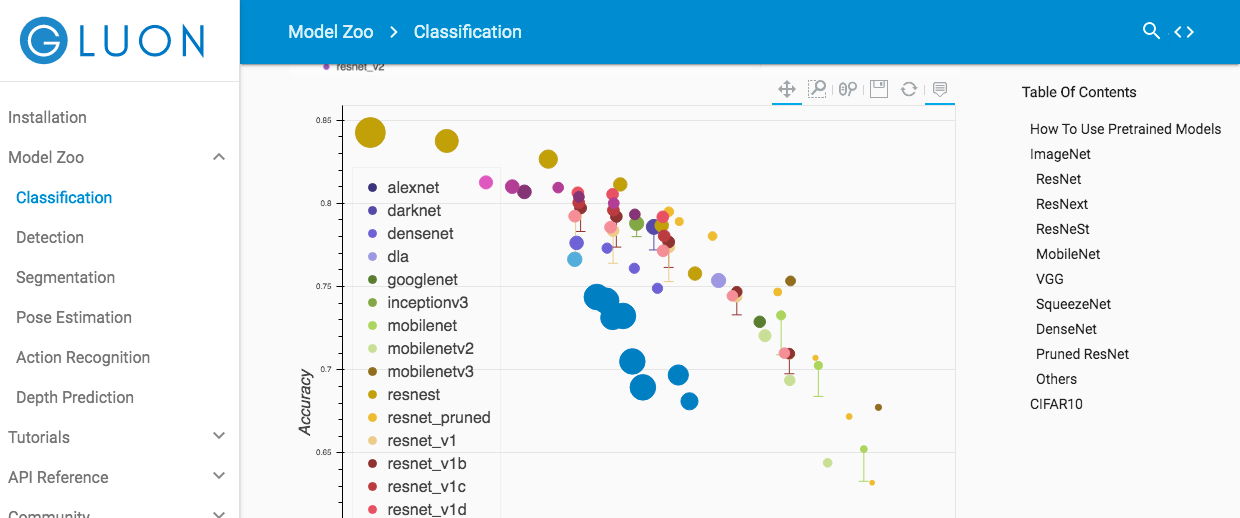# Introduction

I tried the image classification of AutoGluon (https://autogluon.mxnet.io/index.html) which is an AutoML library in the environment of Google Colaboratory. Basically, it will be the content that is added to the content of the official Quick Start.

# environment

It is carried out at Google Colaboratory.

# Run

For image classification, the Deep Learning model is used, so set it to use the GPU (see the figure below).## Library installation on Google Colab

With only the above settings, when you run AutoGluon, you will get an error if you don't have a GPU. Therefore, I installed it referring to this article (https://qiita.com/tasmas/items/22cf80a4be80f7ad458e). There were no particular errors and I was able to do it well.

``````
!pip uninstall -y mkl
!pip install autogluon
!pip install -U ipykernel

``````

Restart the runtime after execution.

## Run Auto Gluon

Follow the official Quick Start. https://autogluon.mxnet.io/tutorials/image_classification/beginner.html

First, import the library and download the data. The data is the image data of Shopee-IET that was in Kaggle, and the images such as clothes are classified into four categories, "Baby Pants", "Baby Shirt", "women casual shoes", and "women chingiffon top". However, the link to Kaggle on the Auto Gluon page was broken. (Although Kaggle also has a page, I couldn't find the data at once. It may have been deleted because it was data from an old competition.)

``````import autogluon as ag
from autogluon import ImageClassification as task

ag.unzip(filename)
``````

Load the training data and the evaluation data respectively.

``````train_dataset = task.Dataset('data/train')
``````

Simply "fit" the training data to build a model for image identification. Insanely easy ...

``````classifier = task.fit(train_dataset,
epochs=5,
ngpus_per_trial=1,
verbose=False)
``````

The following is the standard output result at the time of fit. Apparently I'm bringing ResNet 50. Finally, it also gives you a learning curve. This time I was learning only 5 epochs, so it took only a few minutes.

``````
scheduler_options: Key 'training_history_callback_delta_secs': Imputing default value 60
scheduler_options: Key 'delay_get_config': Imputing default value True

Starting Experiments
Num of Finished Tasks is 0
Num of Pending Tasks is 2
scheduler: FIFOScheduler(
DistributedResourceManager{
(Remote: Remote REMOTE_ID: 0,
<Remote: 'inproc://172.28.0.2/371/1' processes=1 threads=2, memory=13.65 GB>, Resource: NodeResourceManager(2 CPUs, 1 GPUs))
})

100%
2/2 [03:58<00:00, 119.19s/it]
100%|██████████| 55344/55344 [00:01<00:00, 45529.30KB/s]
[Epoch 5] Validation: 0.456: 100%
5/5 [01:06<00:00, 13.29s/it]

Saving Training Curve in checkpoint/plot_training_curves.png
``````The accuracy at the time of learning was about 50%. I think it can't be helped with the current settings.

``````
print('Top-1 val acc: %.3f' % classifier.results['best_reward'])
# Top-1 val acc: 0.469
``````

Let's make a prediction for a certain image data. If you predict the data of "Baby Shirt", it is certainly classified as "Baby Shirt".

``````
image = 'data/test/BabyShirt/BabyShirt_323.jpg'
ind, prob, _ = classifier.predict(image, plot=True)

print('The input picture is classified as [%s], with probability %.2f.' %
(train_dataset.init().classes[ind.asscalar()], prob.asscalar()))

# The input picture is classified as [BabyShirt], with probability 0.61.
``````

When calculating Accuracy using the evaluation data, it was about 70%.

``````test_acc = classifier.evaluate(test_dataset)
print('Top-1 test acc: %.3f' % test_acc)
# Top-1 test acc: 0.703
``````

It's really convenient because you can build a model in a blink of an eye like this, while I felt that the fit function was really important, so I did some research.

## A little research on fit

### Arguments of fit function

This time, I used the fit function in image identification. The source code (https://github.com/awslabs/autogluon/blob/15c105b0f1d8bdbebc86bd7e7a3a1b71e83e82b9/autogluon/task/image_classification/image_classification.py#L63) is excerpted below.

``````
@staticmethod
def fit(dataset,
net=Categorical('ResNet50_v1b', 'ResNet18_v1b'),
optimizer=NAG(
learning_rate=Real(1e-3, 1e-2, log=True),
wd=Real(1e-4, 1e-3, log=True),
multi_precision=False
),
loss=SoftmaxCrossEntropyLoss(),
split_ratio=0.8,
batch_size=64,
input_size=224,
epochs=20,
final_fit_epochs=None,
ensemble=1,
metric='accuracy',
ngpus_per_trial=1,
hybridize=True,
scheduler_options=None,
search_strategy='random',
search_options=None,
plot_results=False,
verbose=False,
num_trials=None,
time_limits=None,
resume=False,
output_directory='checkpoint/',
visualizer='none',
auto_search=True,
lr_config=Dict(
lr_mode='cosine',
lr_decay=0.1,
lr_decay_period=0,
lr_decay_epoch='40,80',
warmup_lr=0.0,
warmup_epochs=0
),
tricks=Dict(
last_gamma=False,
use_pretrained=True,
use_se=False,
mixup=False,
mixup_alpha=0.2,
mixup_off_epoch=0,
label_smoothing=False,
no_wd=False,
teacher_name=None,
temperature=20.0,
hard_weight=0.5,
batch_norm=False,
use_gn=False),
**kwargs):
``````

Because ResNet50 was called by default in `` `net``` which is one of the arguments You can see that it was called earlier. So when you want to try another model, the question is what model you can choose.

### About the model to call

Gluoncv model_zoo (https://gluon-cv.mxnet.io/model_zoo/classification.html) as described on the official page (https://autogluon.mxnet.io/tutorials/image_classification/hpo.html) ) Seems to be able to fetch the model. The registered models as of September 4, 2020 are as shown in the figure below.In addition to ResNet mentioned above, we found that there are some trained models such as MobileNet and VGG. The models are already displayed in order of accuracy, so I think it's easy to choose.

On the other hand, if you want to build your own Neural Network, it seems that you can make it using `` `mxnet``` which is used in the base of AutoGluon.

``````def mnist_net():
mnist_net = gluon.nn.Sequential()
Besides that, you can also set ``metric`` and `optimiser`, so I thought it would be easy to do deep learning.